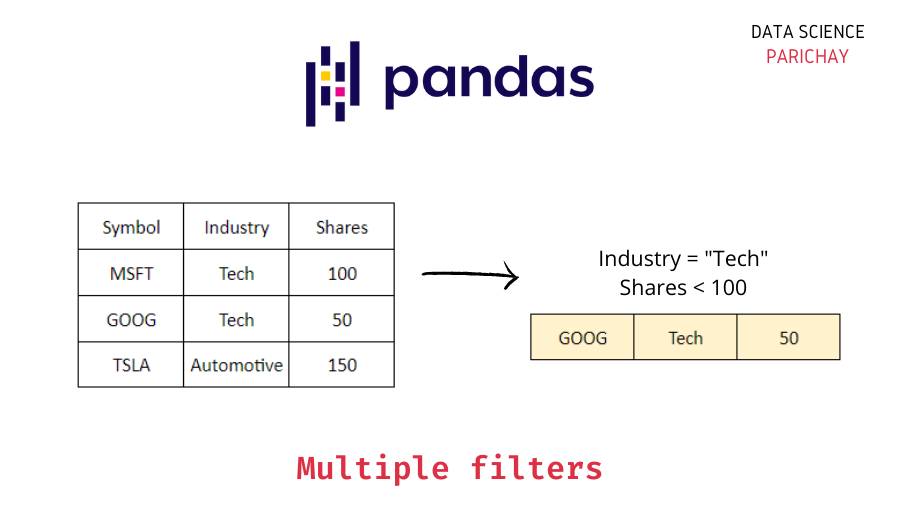# Pandas – Filter DataFrame for multiple conditions

Filtering is one of the most common dataframe manipulations in pandas. When working with data in pandas dataframes, you’ll often encounter situations where you need to filter the dataframe to get a specific selection of rows based on your criteria which may even involve multiple conditions.

In this tutorial, we’ll look at how to filter a pandas dataframe for multiple conditions through some examples. First, let’s create a sample dataframe that we’ll be using to demonstrate the filtering operations throughout this tutorial.

```import pandas as pd

data = {
'Name': ['Microsoft Corporation', 'Google, LLC', 'Tesla, Inc.',\
'Apple Inc.', 'Netflix, Inc.'],
'Symbol': ['MSFT', 'GOOG', 'TSLA', 'AAPL', 'NFLX'],
'Industry': ['Tech', 'Tech', 'Automotive', 'Tech', 'Entertainment'],
'Shares': [100, 50, 150, 200, 80]
}

df = pd.DataFrame(data)
print(df)```

Output:

```                    Name Symbol       Industry  Shares
0  Microsoft Corporation   MSFT           Tech     100
1            Google, LLC   GOOG           Tech      50
2            Tesla, Inc.   TSLA     Automotive     150
3             Apple Inc.   AAPL           Tech     200
4          Netflix, Inc.   NFLX  Entertainment      80```

The sample dataframe `df` stores information on stocks in a sample portfolio.

Pandas dataframes allow for boolean indexing which is quite an efficient way to filter a dataframe for multiple conditions. In boolean indexing, boolean vectors generated based on the conditions are used to filter the data. Multiple conditions involving the operators `|` (for or operation), `&` (for and operation), and `~` (for not operation) can be grouped using parenthesis `()`.

In the sample dataframe created, let’s filter for all the stocks that are in the Tech industry and have 100 or more shares in the portfolio.

```df_filtered = df[(df['Industry']=='Tech')&(df['Shares']>=100)]
print(df_filtered)```

Output:

```                    Name Symbol Industry  Shares
0  Microsoft Corporation   MSFT     Tech     100
3             Apple Inc.   AAPL     Tech     200```

The resulting dataframe after filtering `df`.

📚 Data Science Programs By Skill Level

Introductory

Intermediate ⭐⭐⭐

🔎 Find Data Science Programs 👨‍💻 111,889 already enrolled

Disclaimer: Data Science Parichay is reader supported. When you purchase a course through a link on this site, we may earn a small commission at no additional cost to you. Earned commissions help support this website and its team of writers.

You should keep in mind the following two things when using boolean indexing to filter dataframes for multiple conditions:

Pandas provides operators `&` (for and), `|` (for or), and `~` (for not) to apply logical operations on series and to chain multiple conditions together when filtering a pandas dataframe. If you instead use the python logical operators, it results in an error.

For example, if we filter for stocks having shares in the range of 100 to 150 using `and` we get an error:

```df_filtered = df[(df['Shares']>=100) and (df['Shares']<=150)]
print(df_filtered)```

Output:

```---------------------------------------------------------------------------
ValueError                                Traceback (most recent call last)
<ipython-input-4-dac68abbe005> in <module>
----> 1 df_filtered = df[(df['Shares']>=100) and (df['Shares']<=150)]
2 print(df_filtered)

~\anaconda3\envs\dsp\lib\site-packages\pandas\core\generic.py in __nonzero__(self)
1477     def __nonzero__(self):
1478         raise ValueError(
-> 1479             f"The truth value of a {type(self).__name__} is ambiguous. "
1480             "Use a.empty, a.bool(), a.item(), a.any() or a.all()."
1481         )

ValueError: The truth value of a Series is ambiguous. Use a.empty, a.bool(), a.item(), a.any() or a.all().```

The error occurred because python’s logical operators (`and`, `or`, `not`) are meant to be used with boolean values so when you try to use them with a series or an array, it’s not clear how to determine whether it’s `True` or `False` and hence it results in a `ValueError`.

If you do not use parenthesis `()` to group your conditions, python evaluates the expression based on operator precedence which can give unintended results with operators `&`, `|` and `~`

For example, if we filter for stocks having shares in the range 100 to 150 without using parenthesis we get an error:

```df_filtered = df[df['Shares']>=100 & df['Shares']<=150]
print(df_filtered)```

Output:

```---------------------------------------------------------------------------
ValueError                                Traceback (most recent call last)
<ipython-input-23-545c272b68ba> in <module>
----> 1 df_filtered = df[df['Shares']>=100 & df['Shares']<=150]
2 print(df_filtered)

~\anaconda3\lib\site-packages\pandas\core\generic.py in __nonzero__(self)
1476
1477     def __nonzero__(self):
-> 1478         raise ValueError(
1479             f"The truth value of a {type(self).__name__} is ambiguous. "
1480             "Use a.empty, a.bool(), a.item(), a.any() or a.all()."

ValueError: The truth value of a Series is ambiguous. Use a.empty, a.bool(), a.item(), a.any() or a.all().```

In the above example, the error because in the absence of parenthesis `()`, the expression `df['Shares']>=100 & df['Shares']<=150`
is evaluated as
`df['Shares'] >= (100 & df['Shares']) <= 150`
since the bitwise `&` operator has higher precedence than the comparison operators `>=` and `<=` and is evaluated first.

Boolean indexing is an effective way to filter a pandas dataframe based on multiple conditions. But remember to use parenthesis to group conditions together and use operators `&`, `|`, and `~` for performing logical operations on series.

If we want to filter for stocks having shares in the range of 100 to 150, the correct usage would be:

```df_filtered = df[(df['Shares']>=100) & (df['Shares']<=150)]
print(df_filtered)```

Output:

```                    Name Symbol    Industry  Shares
0  Microsoft Corporation   MSFT        Tech     100
2            Tesla, Inc.   TSLA  Automotive     150```

For more on boolean indexing in pandas, refer to its official documentation.

With this, we come to the end of this tutorial. The code examples and results presented in this tutorial have been implemented in a Jupyter Notebook with a python (version 3.8.3) kernel having pandas version 1.0.5

•